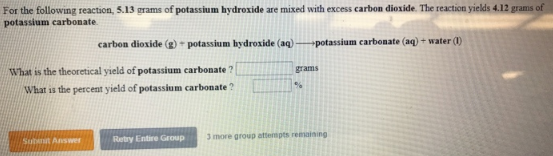# Problem: For the following reaction, 5.13 grams of potassium hydroxide are mixed with excess carbon dioxide. The reaction yields 4.12 grams of potassium carbonate. carbon dioxide (g) + potassium hydroxide (aq) → potassium carbonate (aq) + water (I) What is the theoretical yield of potassium carbonate?What is the percent yield of potassium carbonate?

###### FREE Expert Solution
83% (311 ratings)###### Problem Details

For the following reaction, 5.13 grams of potassium hydroxide are mixed with excess carbon dioxide. The reaction yields 4.12 grams of potassium carbonate.

carbon dioxide (g) + potassium hydroxide (aq) → potassium carbonate (aq) + water (I)

What is the theoretical yield of potassium carbonate?

What is the percent yield of potassium carbonate?